## Abstracts

1. Some sharp bounds for the Euclidean operator radius of two bounded linear operators in Hilbert spaces are given. Their connection with Kittaneh's recent results which provide sharp upper and lower bounds for the numerical radius of linear operators are also established.

2. Some norm inequalities for sequences of linear operators defined on Hilbert spaces that are related to the classical Schwarz inequality are given. Applications for vector inequalities are also provided.

3. New inequalities for the general case of convex functions defined on linear spaces which improve the famous Jensen's inequality are established. Particular instances in the case of normed spaces and for complex and real n-tuples are given. Refinements of Shannon's inequality and the positivity of Kullback-Leibler divergence are obtained.

4. Some new inequalities of the Kantorovich type are established. They hold for larger classes of operators and subsets of complex numbers than considered before in the literature and provide refinements of the classical results in the case when the involved operator satisfies the usual conditions. Several new reverse inequalities for the numerical radius of a bounded linear operator are obtained as well.

5. Some inequalities between the operator norm, numerical radius and the functionals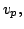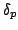defined in terms of the real and imaginary part of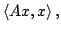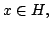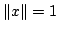are established. New upper bounds for the nonnegative quantity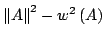with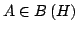that complement some recent results of the author are given as well.

1. By a simple approach, two classes of functions involving Euler's gamma function and originating from certain problems of traffic flow are proved to be logarithmically completely monotonic and a class of functions involving the psi function is showed to be completely monotonic.

2. In this note a procedure to obtain identities involving rational sums of real numbers is presented. As an application, some sums involving binomial coefficients and harmonic numbers are obtained.